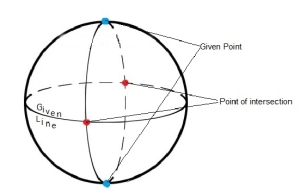## Non Euclidean Geometries

10 Sep 2011

I wrote a few articles on Mathematics for a magazine for school kids, EducationEdge. These articles are targeted at students of classes VIII to XII. Am reproducing one of them here, hoping some of the readers of this blog might enjoy it too.

## Introduction

Science seeks to figure out how the Universe works, and tries to discover the laws which govern it. Mathematics has no such obligations. Though mathematicians and their theories generally stick to the Universe that we live in, once in a blue moon there will rise an eccentric, but genius mathematician, who will propose a radical mathematical theory, which though marvelous, has seemingly no application - at least not in this universe. In this article, we shall talk about Non-Euclidean Geometry, which is literally out-of-this-world Mathematics.

## What is Geometry?

Since we have all studied some geometry in school, we have generally some intuitive idea about what lines, points, circles and planes are. The ancient Greek mathematician Euclid showed that all our intuitive notion about geometric objects can be summarized into a set of five postulates. If these postulates are assumed to be true, all the rest of geometry follows. Hence, the next time your teacher tells you to memorize some rules about circles or parallel lines, you can refuse to do so, saying that you already know Euclid's postulates. (Disclaimer: The author is not responsible for what your teacher does to you after this!!). Euclid's postulates are as follows:

1. A straight line can be drawn that connects two given points
2. A line segment can be extended in both directions to get a straight line
3. A circle with a given center and radius can be drawn
4. All right angles are equal to each other
5. Given a line and a point not lying on it, exactly one line can be drawn that passes through the given point and is parallel to the given line.

## Non-Euclidean Geometry

As you can see, the postulates seem simple and obviously true. However, mathematicians are not simple and obvious creatures. A few mischievous mathematicians thought, "What if we assume one of these postulates to be false? What if we assume something that is contrary to one of these postulates to be true?'' They did so, and came up with several different kinds of geometries, all of which are inconsistent with our normal notion of Geometry, but nonetheless, consistent within themselves. "But what is the use of all this?'', you may ask, "If these geometries are not real, and don't work in our Universe at all, why do mathematicians want to study them?'' These are very valid questions, but mathematicians are a crazy bunch of people, and often do mathematics just for the sake of it, even if it seems to have absolutely no utility anywhere in the real world.

## Elliptical Geometry

One of the non-Euclidean geometries, that is relatively easy to understand is called Elliptical Geometry. In this geometry, the fifth postulate in Euclid's postulates is changed.

• Given a line and a point not lying on it, no line can be drawn that passes through the given point and is parallel to the given line.

In order to understand this, you must suspend your usual notions about what a point, line, etc are. In our new universe, these are entirely different things than what we usually think of them. We shall hence redefine them in our new universe. To avoid confusion, we shall write point when we wish to refer to constructs in the new universe, and point when we wish to refer to our usual notion. Imagine a sphere. A plane is defined as the surface of this sphere. A point is defined as a pair of diametrically opposite points on this sphere. A line is a great circle on the sphere(A great circle is a circle, like the equator, whose plane contains the center of the sphere). Notice that two lines always intersect in exactly two diametrically opposite points, that is exactly one point.Observe that in this elliptical geometry, the first four of Euclid's postulates still hold. (Keep down this article and think about the first two postulates now. I assure you that you will find it a rewarding exercise). Though we have not formally defined circles and angles due to lack of space, it can be proved that the third and fourth postulates also hold true in elliptical geometry. Notice also, that the new fifth postulate is now true in this system. Given a line and a point lying outside it, it is impossible to construct a line parallel to the one given. Remember that a line must lie on the plane and since, in this case a plane is the surface of the sphere, the plane of any line through a given point must pass through the centre of the sphere and thus must intersect the given line at some point (see Figure). This makes it impossible, in Elliptical Geometry, to draw a pair of parallel lines! Many other interesting and non-intuitive facts also emerge in this system. For example, the sum of the angles of a triangle is greater than 180 degrees. Mindboggling, but true.

## Conclusion

We saw that even a simple subject like geometry is a subject of deep mathematical study. The essence of mathematics is to question. Mathematicians ask questions like, What is a number?'' or What is a point?'' Though they seem silly, finding the answers to these questions involves much thought, and a journey full of adventure into the dark and mysterious land of mathematics.